Click to Chat

1800-1023-196

+91-120-4616500

CART 0

• 0

MY CART (5)

Use Coupon: CART20 and get 20% off on all online Study Material

ITEM
DETAILS
MRP
DISCOUNT
FINAL PRICE
Total Price: Rs.

There are no items in this cart.
Continue Shopping• Complete Physics Course - Class 11
• OFFERED PRICE: Rs. 2,968
• View Details

Kinetic Energy

Table of Content

Energy (E)

Mechanical Energy

Definition of Kinetic Energy

Mathematical Expression

Relation Between Kinetic Energy (K) and Momentum (p)

Work-Energy Theorem

Conservation of Energy

Some Conceptual Question

Related Resources

Energy (E)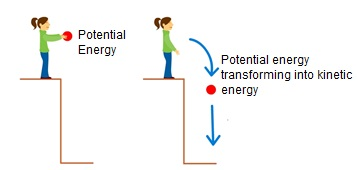It is the ability of the body to do some work. The unit of energy is same as that of work.

Greater the amount of energy contained in the body, greater work it will able to do. In our daily life we see so many examples where bodies are able to do work due to one reason or the other.

(a) A speedy bullet is able to penetrate a block of wood , thus, doing work against friction.

(b) A fast blowing wind is able to turn a wheel.

(c)  A fan statrs rotating when electricity is fed to it.

All the agents which are able to do work are said to be energetic. we shall come across various types of energies during the study of Physics, for examples-mechanicical energy, heat energy, light energy, electric energy etc. For the time being we shall concentrate on the mechanical energy only.

Mechanical Energy

The energy possessed by a body by virtue of its motion or by virtue of its position and/or configuration is called mechanical energy.

Mechanical energy is of two types:-

(a) Kinetic energy

(b) Potential energy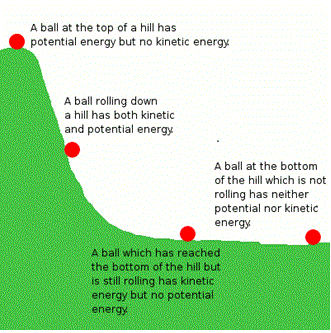Definition of Kinetic Energy

It has been observed that a high speed bullet is able to pierce a block of wood, thus, doing work against friction.

Energy possessed by a body by virtue of its motion is called kinetic energy. Thus the kinetic energy is the form of energy contained in an object’s motion.

The side figure shows the change in kinetic energy of the ball at the different position of a hill. The energy just tranforms from one part to the other part. So the total energy of the system remains conserved.

Mathematical Expression

Consider a body of mass ‘m’ rolling over a rough horizontal surface. Its velocity while it crosses ‘A’ is V and at ‘B’ is zero as shown in the below figure. In going from A to B, force of friction opposes the motion. The body has to do work against friction. It means that work done by the body in going from A to B must be equal to the initial amount of energy (kinetic energy), contained in the body, while at A.

If  a is the acceleration of the body, then the force of friction F will be F = ma.

Let ‘W’ be the work done by the body is going from A to B.

= FS cos 180º

W = -FS   (Since, cos 180º = -1)

So,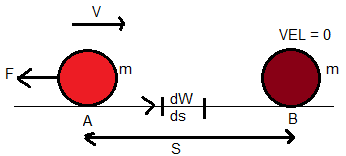W = -mas

Initial velocity at A, u = V        Final velocity at B, v = 0

Acceleration = a                     Distance travelled = S

Applying the relation,

v2 – u2 = 2aS

Or, 0 – V2 = 2aS

So, a = – (V2/2S)

Substituting for ‘a’ in equation (5),

W = -m (-V2/2S) S

Or, W = ½ mV2

Since K.E. of the body is equal to the work done by the body, therefore,

K.E = ½ mV2

Alternative Method:-

Let dW = work done by the body in moving through a distance ‘dS’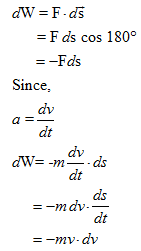Integrating both sides, we can get total work done,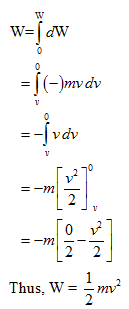Since K.E. is equal to the work done,Relation Between Kinetic Energy (K) and Momentum (p)

Kinetic energy,

K = ½ mv2

= ½ (m2v2/m)

but, mv = p (momentum of body)

Or, K = p2/2m

(a) If p = constant,          K∝(1/m)

If two bodies of different masses have same momentum, body with a greater mass shall have lesser kinetic energy.

(b) If K = constant,          p∝√m

If two bodies of different mass have same kinetic energy, body with a greater mass shall have greater momentum.

(c)  If m = constant,       K∝p2

For two bodies having same mass, the body having greater momentum shall have greater kinetic energy.

Work-Energy Theorem

“The net work done (Wnet) by the forces acting on a body is equal to the change in the kinetic energy of the body”.

So, Wnet = ½ mvf2 – ½ mvi2

= Kf – Ki

Conservation of Energy

Law of conservation of energy was first stated by Robert Mayor in 1842.

It states that, “Energy can neither be created nor destroyed. It can be converted from one form to another. The sum of total energy, in this universe, is always same”.The sum of the kinetic and potential energies of an object is called mechanical energy.

So, E = K+U

In accordance to law of conservation of energy, the total mechanical energy of the system always remains constant.

So, mgh + ½ mv2 = constant

In the side figure, the kinetic and potentail energy of a simple pendulum is given. The K.E and P.E will be different at the different , different position of the bob. The loss of kinetic enegy will be eqaul to the gain in potential energy. So the energy just goes from one form to other. This signifies, the total mecahnical energy (TME) of the system will be conserved.

Refer to the following video for “all forms of energy” and “law of conservation of energy”:-

Some Conceptual Question

Question 1:-

Does kinetic energy depend on the direction of the motion involved? Can it be negative? Does its value depend on the reference frame of the observer?

No, kinetic energy does not depend on the direction of motion involved. Kinetic energy can never be negative. Yes, the value of kinetic energy depends on the reference frame of the observer.

Kinetic energy (K) of a particle is defined as the,

K = ½ mv2

Where m is the mass of the particle and v is the velocity of the particle.

Since kinetic energy is a scalar quantity, therefore there is no direction associated with kinetic energy and it has no components. Thus kinetic energy does not depend on the direction of motion involved.

Since, mass of the body (m) is always a positive quantity and the term v2 in the kinetic energy is always positive, therefore, kinetic energy of a body is always a positive quantity. Therefore kinetic energy can never be negative.

Newton’s laws are valid only in inertial frames of reference. If we find Newton’s second law to hold in one frame of reference, then it holds in all inertial frames. If two observers in different inertial frames move at constant velocity v relative to one another and observe the same experiment, they measure identical values for the forces, masses, and accelerations, and so they agree completely in their analysis using Newton’s second law.

In the Newton’s second law, observers in different inertial frames will agree on the results of applying the work energy theorem. However, unlike forces and accelerations, displacements and velocities measured by observers in different inertial frames will in general be different, and so they will deduce different values for the work and kinetic energies in the experiment. Therefore the value of kinetic energy depends on the reference frame of the observer.

Question 2:-

Does the work-energy theorem hold if friction acts on an object? Explain your answer.

No, the work-energy theorem does not hold if friction acts on an object.

Work-Energy theorem states that, the net work done by the forces acting on a body is equal to the change in the kinetic energy of the body. In a frictional surface we do not count the part of work which will convert into the friction. The frictional force is in reality quite complicated, involving the making and breaking of many microscopic welds, which deform the surfaces and result in changes in internal energy of the surfaces (which may in part be revealed as an increase in the temperature of the surfaces).So the work done by the force acting on the body is not totally converted into its kinetic energy and that contradicts the work energy theorem. From the above observation we conclude that, the work-energy theorem does not hold if friction acts on an object.

Question 3:-

Why can a car so easily pass a loaded truck when going up hill? The truck is heavier, of course, but its engine is more powerful in propertion (or is it)?. What considerations enter into choosing the design power of a truck engine and of a car engine?

In going up-hill by an object, energy is required. The amount of energy depends on the mass of the object. Thus, the object having heavier mass required more energy to move uphill than the lighter one.

As the mass of the car is lesser than the mass of the loaded truck, lesser amount of energy is required by the car to move uphill. On the other hand, large amount of energy is required by the loaded truck to move uphill. Therefore, a car can easily pass a loaded truck when going uphill.

To design power of a truck engine and a car engine, the material should light to decrease the weight of the body. Therefore lesser amount of energy will be required to move the body uphill.

Problem 1:-

A single force acts on a particle in rectilinear motion. A plot of velocity versus time for the particle is shown in below figure. Find the sign (positive or negative) of the work done by the force on the particle in each of the intervals AB, BC, CD, and DE.Concept:-

The net work done (Wnet) by the forces acting on a body is equal to the change in the kinetic energy of the body.

So, Wnet = ½ mvf2 – ½ mvi2

Here m is the mass of the body, vi is the initial velocity of the body and vf is the final velocity of the body. If the kinetic energy of the particle is decreasing in a certain region then the work done by the force on the particle will be negative and if the kinetic energy of the particle is increasing in a certain region then the work done by the force on the particle will be positive.

Solution:-

A single force acts on a particle in rectilinear motion. A plot of velocity versus time for the particle is shown in the below figure.

In the interval AB, change in kinetic energy of the particle is positive. So kinetic energy of the particle is increasing, therefore the sign of the work done will be positive.

In the interval BC, change in kinetic energy of the particle is zero since initial velocity (vi) of the particle is equal to the final velocity (vf) of the particle in the interval BC. Therefore in this region work done will be zero.

In the interval CD, change in kinetic energy of the particle is negative. So kinetic energy of the particle is decreasing, therefore the sign of the work done will be negative.

In the interval DE, change in kinetic energy of the particle is positive. So kinetic energy of the particle is increasing, therefore the sign of the work done will be positive.

Problem 2:-

Calculate the kinetic energies of the following objects at the given speeds; (a) a 110-kg football linebacker running at 8.1 m/s; (b) a 4.2 bullet at 950 m/s; (c)  the aircraft carrier (91,400 tons at 32.0 knots)

Concept:-

Kinetic energy K of a body having mass m moving with speed v is equal to the ½ mv2.

So, K = ½ mv2       …… (1)

Solution:-

(a) We have to find out the kinetic energy of the football having mass 110-kg and at a speed of 8.1 m/s.

To obtain Kinetic energy K of the football, substitute 110-kg for mass m and 8.1 m/s for speed the football v in the equation K = ½ mv2,

K = ½ mv2

= ½ (m) (v)2

= ½ (110-kg) (8.1 m/s)2

= 3608.55 kg. m2/s2

= (3608.55 kg. m2/s2) (1 J/1 kg. m2/s2)

= 3608.55 J     …… (2)

Rounding off to two significant figures, the kinetic energy of the football will be 3600 J.

(b) We have to find out the kinetic energy of the bullet having mass 4.2-g and at a speed of 950 m/s.

To obtain Kinetic energy K of the bullet, substitute 4.2-g for mass m and 950 m/s for speed the bullet v in the equation K = ½ mv2,

K = ½ mv2

= ½ (m) (v)2

= ½ (4.2-g) (950 m/s)2

= ½ ((4.2-g) (10-3 kg/1 g)) (950 m/s)2

= ½ (4.2×10-3 kg) (950 m/s)2

=1895.25 kg. m2/s2

= (1895.25 kg. m2/s2) (1 J/1 kg. m2/s2)

= 1895.25 J     …… (3)

Rounding off to two significant figures, the kinetic energy of the bullet will be 1900 J.

(c)  We have to find out the kinetic energy of the aircraft having mass 91,400 tons and at a speed of 32.0 knots.

To obtain Kinetic energy K of the aircraft, substitute 91,400 tons for mass m and 32.0 knots for speed the aircraft v in the equation K = ½ mv2,

K = ½ mv2

= ½ (m) (v)2

= ½ (91,400 tons) (32.0 knots)2

= ½ ((91,400 tons) (103 kg/1 ton)) ((32.0 knots)(0.5144 m/s / 1 knot))  2

= ½ (914×105 kg) (16.46 m/s)2

=1.24×1010 kg. m2/s2

= (1.24×1010 kg. m2/s2) (1 J/1 kg. m2/s2)

= 1.24×1010 J     …… (4)

From the equation (4) we observed that, the kinetic energy of the aircraft will be 1.24×1010 J.

Problem 3:-

A proton (nucleus of the hydrogen atom) is being accelerated in a linear accelerator. In each stage of such an accelerator the proton is accelerated along a straight line at 3.60×1015 m/s2. If a proton enters such a stage moving initially with a speed of  2.40×1015 m/s and the stage is 3.50 cm long, compute (a) its speed at the end of the stage and (b) the gain in kinetic energy resulting from the acceleration. The mass of the proton is 1.67×10-27 kg. Express the energy in electron volts.

Concept:-

Kinetic energy K of a body having mass m moving with speed v is equal to the ½ mv2.

So, K = ½ mv2    …… (1)

From equation (1), speed of the particle will be,

v = √2K/m       …… (2)

Work done (W) by a force F that moves a body through a displacement x will be,

W = Fx          …… (3)

Again in accordance to work energy theorem, the net work done by the forces acting on a body is equal to the change in the kinetic energy ΔK of the body.

So, ΔK = Kf- Ki

= W           …… (4)

From equation (3) and (4) we get,

ΔK = W

= Fx

= max           (Since, F = ma) …… (5)

Solution:-

(a) To obtain the final speed vf of the proton, first we have to find out the final kinetic Kf energy of the proton.

To obtain the change in the kinetic energy ΔK, substitute 1.67×10-27 kg for mass of the proton m, 3.60×1015 m/s2 for a and 3.50 cm for x in the equation  ΔK = max, we get,

ΔK = max

= (1.67×10-27 kg) (3.60×1015 m/s2)( 3.50 cm)

= (1.67×10-27 kg) (3.60×1015 m/s2)( 3.50 cm×10-2 m/1 cm)

=(2.10×10-13 kg. m2/s2) (1 J/1 kg. m2/s2)

= (2.10×10-13 J) (1 eV/1.6×10-19 J)

= 1.31×106 eV

So, Kf- Ki = 2.10×10-13 J (Since, ΔK = Kf- Ki)

Or, Kf = (2.10×10-13 J) + Ki

= (2.10×10-13 J) + ½ mvi2   (Since, Ki =½ mvi2 )

= (2.10×10-13 J) + ½ (1.67×10-27 kg) (2.40×107 m/s2)2

= [2.10×10-13 J] +[(4.81×10-13 kg. m2/s2) (1 J/1 kg. m2/s2)]

= [2.10×10-13 J] +[4.81×10-13 J]

=6.91 ×10-13 J

To obtain the final speed vf of the proton, substitute 6.91 ×10-13 J for Kf and 1.67×10-27 kg for mass of the proton m in the equation v = √2Kf/m, we get,

v = √2Kf/m

=√2 (6.91 ×10-13 J) /(1.67×10-27 kg)  (1 kg. m2/s2/ 1 J)

=2.88×107 m/s

From the above observation we conclude that, the final speed vf of the proton would be 2.88×107 m/s.

(b) To find out the speed of proton at the end of the stage, we have to find out the change in the kinetic energy ΔK.

To obtain the change in the kinetic energy ΔK, substitute 1.67×10-27 kg for mass of the proton m, 3.60×1015 m/s2 for a and 3.50 cm for x in the equation  ΔK = max, we get,

ΔK = max

= (1.67×10-27 kg) (3.60×1015 m/s2)( 3.50 cm)

= (1.67×10-27 kg) (3.60×1015 m/s2)( 3.50 cm×10-2 m/1 cm)

=(2.10×10-13 kg. m2/s2) (1 J/1 kg. m2/s2)

= (2.10×10-13 J) (1 eV/1.6×10-19 J)

= 1.31×106 eV

From the above observation we conclude that, the speed of proton at the end of the stage would be 1.31×106 eV.

Related Resources

Look into the Sample Papers of Previous Years to get a hint of the kinds of questions asked in the exam.

You can get the knowledge of Useful Books of Physics.### Course Features

• 101 Video Lectures
• Revision Notes
• Previous Year Papers
• Mind Map
• Study Planner
• NCERT Solutions
• Discussion Forum
• Test paper with Video Solution